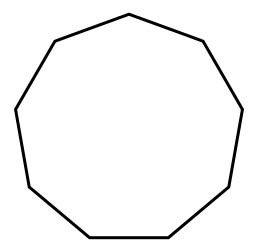# Swift Program to Calculate the Area of Enneagon

This tutorial will discuss how to write swift program to calculate area of enneagon.

A enneagon is a polygon with 9 straight sides and 9 vertices. The sum of all the interior angles is 1260 degree. The total amount of space enclosed inside the nine sides of the enneagon is known as the area of the enneagon.## Formula

Following is the formula of the area of the enneagon −

Area = ((x2*9) / 4*tan (20°)) ∼= (6.18 * x2)


Here, x is the side of the enneagon.

Below is a demonstration of the same −

Input

Suppose our given input is −

side = 4


Output

The desired output would be -

Area of the enneagon = 98.88


## Algorithm

Following is the algorithm-

Step 1 - Create a function with return value.

Step 2 - Find the area of the enneagon using the following formula −

let result = 6.18 * side * side


Step 3 - Calling the function and pass the side in the function as a parameter.

Step 4 - Print the output.

## Example

The following program shows how to calculate the area of enneagon.

import Foundation
import Glibc
// Creating a function to calculate area of the enneagon
func enneTriangle(side: Double){
let result = 6.18 * side * side
print("Area of the enneagon: \(result)")
}

// Side of the enneagon
var x = 10.0
print("Side: \(x)")

// Calling function
enneTriangle(side:x)


## Output

Side: 10.0
Area of the enneagon: 618.0


Here, in the above program we create a function which return the area of the enneagon using the following formula −

let result = 6.18 * side * side


Here, the side of enneagon is 10 so the area is 618.0

Updated on: 30-Nov-2022

89 Views